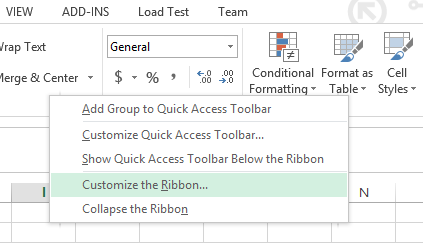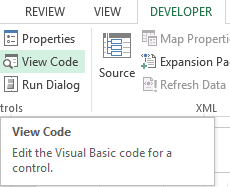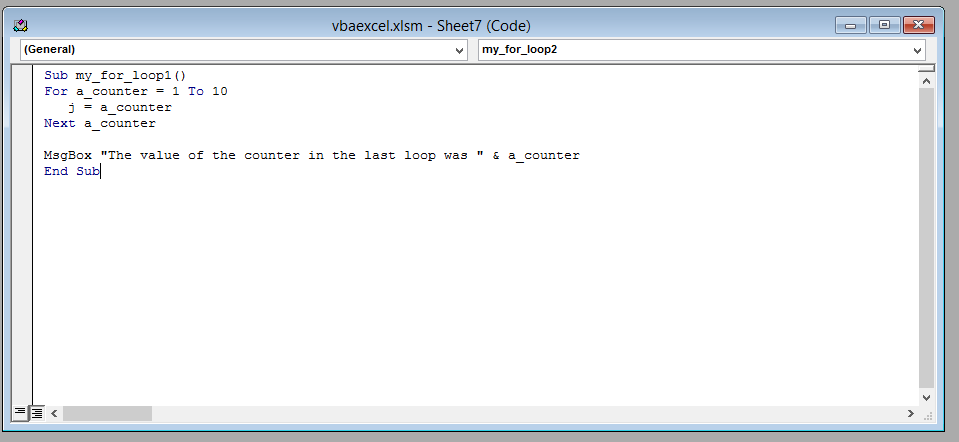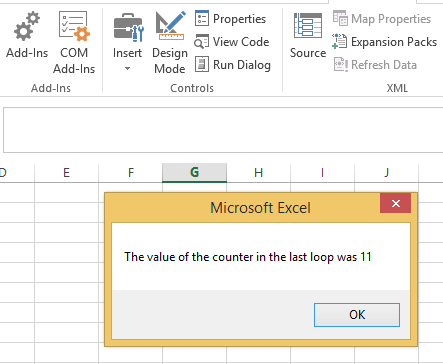## For Next Loop

In this article we are going to learn about programming FOR NEXT loops. First we are going to learn what loops are and why they are necessary. Then we are going to learn how to use a FOR NEXT LOOP.

Note: In excel VBA they are two types of FOR LOOPS: FOR NEXT and FOR EACH NEXT. Our focus will be on the FOR NEXT loop in this article.Consider the following code:

```Dim numbcount As Integer
numbcount = 1 + 2 + 3 + 4 + 5 + 6 + 7 + 8 + 9 + 10```

This code is used to add the numbers from 1 to 10. The result is then stored in the variable numbcount. Now this looks pretty easy and simple right. But now suppose instead of the first ten number you want to add numbers 1 to 1000, now are you going to write all the numbers and then add them? Of course you can but that would become tedious right. So what do we do? It’s easy actually we use the handy programming tool called “Loop”.

Loop as the names sound is something that goes on and on until you tell it to stop (this is called a “condition”). You set a starting number for your loop, an end condition, and a way to get from the starting number to the end condition. In VBA, there are four types of loop to choose from: For Next loops, For Each Next loop, Do Loops, and While loops. We will focus on the FOR NEXT loop for now.

First let’s look at the syntax of the FOR NEXT LOOP:

```For a_count = start_count To end_counter
'Do something here
Next a_count```

Explanation: a_count is the variable name. a_count will have the values of start_count and end_count.The “Do something here” is the body of the for loop where you write what you want the FOR NEXT loop to do.

Here is an example for you to understand the syntax better:

```Sub myforloop()
Dim j As Integer
For a_counter = 1 To 10
j =a_count
Next a_count```

Explanation: In this code we are creating a For Next loop that uses a variable a_count as the “time keeper” of the loop. We set value of a_count to the value of start_counter at the beginning of the loop and then increment it by 1 during each loop till. The loop will keep on executing until the time the value of a_count becomes equal to end_counter. The “Do something here” is the body of the loop. Here we write what we want the loop to do.

Note: The final value of the a_count in the above loop will be 11

Now let’s see how to use the For Next loop. Follow the steps below:

STEP-1 Right click anywhere on the ribbon and select “Customize the Ribbon” from the drop down menu.STEP-2 An option dialog box will open. Click on the DEVELOPER check box (it is under “Customize the Ribbon Main Tabs”) and press ok.STEP-3 The developer tap is now visible and is present next to the view tab on the top menu bar. Click on Developer tab and select “View Code”STEP-4 A new window (Visual Basic Editor) will open which would have a dialog box in the center. You will write the code in the dialog box.STEP-5 Write the following line of code in the dialog box.

```Sub my_for_loop1()
For a_count = 1 to 10
j= a_count
Next a_count
MsgBox "The value of the counter in the last loop was" & a_count
End Sub```

After writing the code close the window by clicking on the cross(x) icon on the upper right side of the screen. Don’t worry Excel won’t close.STEP-6 This is the result. That’s it! You have successfully used a For Next loop.## Template

```Further reading: